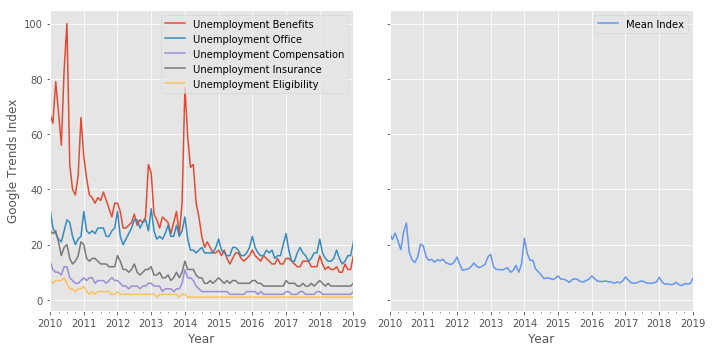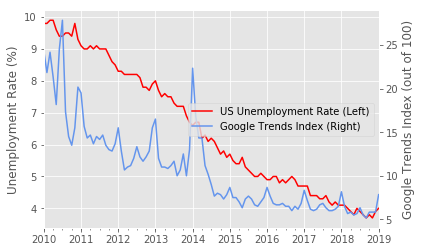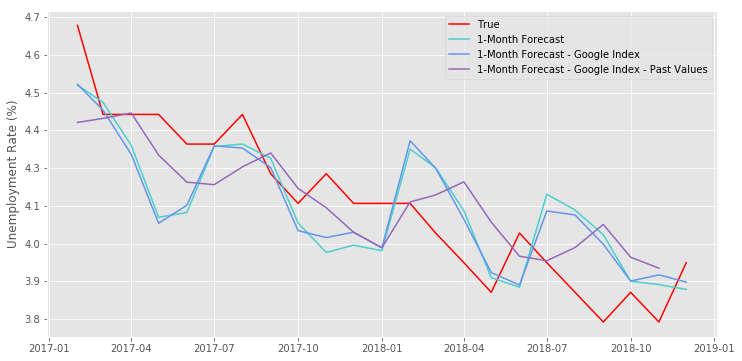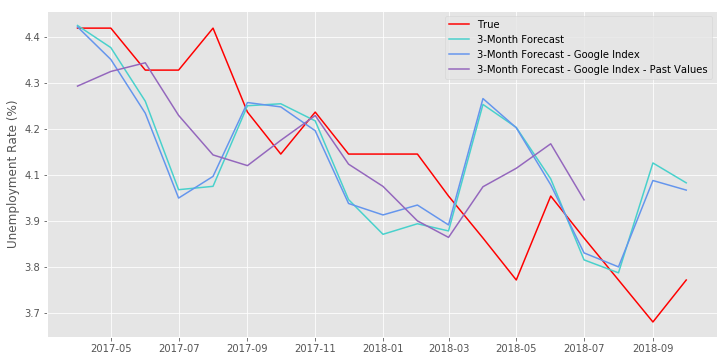# Using Google Trends Index and High-frequency State-level Data to Predict National Unemployment Rates¶

A common issue with macro-economic data is that it is reported at low frequencies, generally quarterly: GDP and unemployment data are the most prominent examples of such macroeconomic indicators. However, policy makers, financial institutions and investors are often interested in having more frequent data on these indicators to be able to make more informed decisions in their respective fields.

In this exercise, I will focus on unemployment rates specifically. Previous literature has forecasted unemployment rates using either structural models (such as DSGE models), or non-structural ones which make use of some econometric tools (such as VAR models) to create predictive models. In a 2017 paper, CH17 use multiple neural network architectures to predict civilian unemployment rates in the US using solely the unemployment rate series. The neural networks that CH17 use are LSTM, Convolutional, Fully Connected and Encoder-Decoder.

Instead, I use a simpler architecture, Multi-Layer Perceptron, and expand their research by using the following data:

• State-level monthly unemployment data from the Bureau of Labour Statistics. This data is retrieved using the Quandl API, and is expressed in percentages.
• Google Trends Index for unemployment-related keywords. This data is expressed as a number from 1 to 100, where 100 represents the popularity of the keyword relative to other word searches within a period and a given location.

The Google trends method for predicting unemployment is inspired by the The Research Institute of the Finnish Economy, which uses unemployment-related keywords to make nowcasts of European unemployment rates (KU16).

To test whether the Google trends data truly has any predictive power, I first run a model with state-level unemployment data which predicts the US national unemployment rate. Then, I use a model which includes Google trends index results for every US state. Lastly, in model 3 I include present and past months of state unemployment and Google Index data to make predictions.

Findings:

1. Using Google Trends data reduces the mean squared error (MSE) at every forecast horizon (1-, 2-, and 3- month).
2. Adding past and present values of state unemployment and Google Trends data to the model considerably improves the prediction.

[CH17] Thomas R. Cook and Aaron Smalter Hall (2017) Macroeconomic Indicator Forecasting with Deep Neural Networks.

[KU16] Tuhkuri, Joonas (2016) ETLAnow: A Model for Forecasting with Big Data – Forecasting Unemployment with Google Searches in Europe.

### Unemployment Data¶

First, I use Quandl's API to download monthly unadjusted unemployment rate data for each US state since 2010. I choose January 2010 as my starting data because Quandl's access to Bureau of Labour Statistics data is restricted to 2010-present.

In :
%%capture
!pip install quandl
import quandl

In :
# Get US national monthly unadjusted unemployment rate data
quandl.ApiConfig.api_key = '2BYtKNrxRuVRhzkA9_2s'
unrate_us = quandl.get('FRED/UNRATE', start_date='2010-01-01', end_date='2019-04-01')
unrate_us.columns = ["US"]
unrate_us.index = unrate_us.index.strftime("%Y-%m")

In :
# Second, we get the monthly state unemployment data
codes_states = ['01', '02', '04', '05', '06', '08', '09', '10', '11', '12',
'13', '15', '16', '17', '18', '19', '20', '21', '22', '23',
'24', '25', '26', '27', '28', '29', '30', '31', '32', '33',
'34', '35', '36', '37', '38', '39', '40', '41', '42', '44',
'45', '46', '47', '48', '49', '50', '51', '53', '54', '55',
'56']
list_states = ("Alabama, Alaska, Arizona, Arkansas, California, "
"Colorado, Connecticut, Delaware, District of Columbia, "
"Florida, Georgia, Hawaii, Idaho, Illinois, Indiana, Iowa, "
"Kansas, Kentucky, Louisiana, Maine, Maryland, Massachusetts, "
"Michigan, Minnesota, Mississippi, Missouri, Montana, Nebraska, "
"Nevada, New Hampshire, New Jersey, New Mexico, New York, North Carolina, "
"North Dakota, Ohio, Oklahoma, Oregon, Pennsylvania, Rhode Island, "
"South Carolina, South Dakota, Tennessee, Texas, Utah, Vermont, Virginia, "
"Washington, West Virginia, Wisconsin, Wyoming").split(", ")

api_codes = ['BLSE/LAUST{}0000000000003'.format(x) for x in codes_states]
quandl.ApiConfig.api_key = '2BYtKNrxRuVRhzkA9_2s'
unrate_states = quandl.get(api_codes, start_date='2004-01-01', end_date='2019-04-01')
unrate_states.columns = list_states
unrate_states.index = unrate_states.index.strftime("%Y-%m")

In :
# Merge the two datasets
unrate = unrate_us.merge(unrate_states, left_index = True, right_index = True)
unrate.tail()

Out:
US Alabama Alaska Arizona Arkansas California Colorado Connecticut Delaware District of Columbia Florida Georgia Hawaii Idaho Illinois Indiana Iowa Kansas Kentucky Louisiana Maine Maryland Massachusetts Michigan Minnesota Mississippi Missouri Montana Nebraska Nevada New Hampshire New Jersey New Mexico New York North Carolina North Dakota Ohio Oklahoma Oregon Pennsylvania Rhode Island South Carolina South Dakota Tennessee Texas Utah Vermont Virginia Washington West Virginia Wisconsin Wyoming
2018-11 3.7 3.3 6.4 4.7 3.2 3.9 3.5 3.1 3.0 5.1 3.3 3.4 2.7 2.7 4.0 3.3 1.9 3.0 3.5 4.3 3.3 3.4 2.6 3.4 2.3 4.2 2.5 3.6 2.3 4.2 2.2 3.3 4.8 3.5 3.6 2.1 4.2 2.9 4.0 3.6 3.9 3.0 2.8 3.0 3.5 2.8 2.4 2.5 4.4 4.6 2.5 4.0
2018-12 3.9 3.5 6.5 4.9 3.6 4.1 3.8 3.2 3.1 4.9 3.3 3.7 2.3 2.9 4.4 3.4 2.5 3.1 3.8 4.4 3.4 3.5 2.7 4.1 3.2 4.7 3.1 3.9 2.7 4.3 2.1 3.6 4.8 3.9 3.7 2.4 4.8 3.0 4.3 3.9 3.8 3.3 3.0 3.0 3.6 2.9 2.3 2.6 4.8 4.9 2.8 4.0
2019-01 4.0 4.4 7.4 5.7 4.5 4.8 4.2 4.7 3.7 6.0 3.9 4.5 2.9 4.1 5.2 4.1 3.3 3.8 4.5 5.2 4.3 4.3 3.6 4.6 4.3 5.2 3.8 5.0 3.1 4.6 2.9 4.6 5.5 4.6 4.5 3.0 5.4 3.8 5.0 4.2 4.9 3.6 3.3 3.7 4.2 3.2 2.8 3.2 5.3 6.0 3.5 4.5
2019-02 3.8 4.0 7.5 4.7 4.3 4.4 3.5 4.4 3.4 6.0 3.4 3.9 2.8 3.7 4.7 4.1 2.7 3.7 4.3 4.4 3.9 4.1 3.2 4.1 4.0 4.8 3.7 4.4 3.1 4.1 2.9 4.7 5.2 4.4 4.2 3.0 4.7 3.5 4.7 4.2 4.3 3.3 3.3 3.2 3.9 3.1 2.5 3.2 5.2 6.1 3.3 3.8
2019-03 3.8 3.8 7.2 4.6 3.4 4.6 3.0 4.2 3.2 5.6 3.3 3.7 2.8 3.4 4.5 3.9 2.8 3.7 4.4 3.9 3.8 3.9 3.1 4.4 4.1 4.8 3.9 4.1 3.2 3.7 2.8 4.1 4.6 4.1 4.1 3.0 4.1 3.2 4.6 3.9 3.7 3.3 3.1 3.3 3.5 3.0 2.3 3.0 5.2 5.3 3.3 3.8
In :
# API library retrieved from: https://github.com/GeneralMills/pytrends
%%capture
!pip install pytrends # --upgrade #--user git+https://github.com/GeneralMills/pytrends
from pytrends.request import TrendReq
pytrends = TrendReq(hl='en-US', tz=360)

In :
# I use the top 5 most popular terms (extracted from the list of 13 most
# searched Google phrases as shows in Tuhkuri(2016) )

keywords = ('unemployment benefits, unemployment office, '
'unemployment compensation, unemployment insurance, '
'unemployment eligibility').split(', ')

pytrends.build_payload(kw_list = keywords, geo = 'US', timeframe = '2010-01-01 2019-01-31')
us = pytrends.interest_over_time()
us_mean = us.mean(axis = 1)
us_mean = us_mean.to_frame(name = 'US')

In :
import matplotlib.pyplot as plt
plt.style.use('ggplot')

fig, ax = plt.subplots(nrows = 1, ncols = 2, figsize = (10,5), sharey = True)
us.plot(ax = ax)
us_mean.plot(ax = ax, label = 'Mean Index', color = 'cornflowerblue')
ax.legend(['Unemployment Benefits', 'Unemployment Office', 'Unemployment Compensation',
'Unemployment Insurance', 'Unemployment Eligibility'])
ax.set_xlabel('Year')
ax.legend(['Mean Index'])
ax.set_xlabel('Year')

plt.tight_layout()In :
# Now, I use the mean index for each US state, and merge the time series into one dataset

state_codes = ["AL", "AK", "AZ", "AR", "CA", "CO", "CT", "DC", "DE", "FL", "GA",
"HI", "ID", "IL", "IN", "IA", "KS", "KY", "LA", "ME", "MD",
"MA", "MI", "MN", "MS", "MO", "MT", "NE", "NV", "NH", "NJ",
"NM", "NY", "NC", "ND", "OH", "OK", "OR", "PA", "RI", "SC",
"SD", "TN", "TX", "UT", "VT", "VA", "WA", "WV", "WI", "WY"]
state_list = ['US-{}'.format(x) for x in state_codes]

for i,j in enumerate(state_list):
pytrends.build_payload(kw_list = keywords, geo = state_list[i], timeframe = '2010-01-01 2019-01-31')
state = pytrends.interest_over_time()
state_mean = state.mean(axis = 1)
state_mean = state_mean.to_frame(name = j)


In :
# Merge the google index data to the unemployment data
import pandas as pd
pd.options.display.max_columns = None

data = unrate.merge(google_index, left_index = True, right_index = True)

Out:
US_x Alabama_x Alaska_x Arizona_x Arkansas_x California_x Colorado_x Connecticut_x Delaware_x District of Columbia_x Florida_x Georgia_x Hawaii_x Idaho_x Illinois_x Indiana_x Iowa_x Kansas_x Kentucky_x Louisiana_x Maine_x Maryland_x Massachusetts_x Michigan_x Minnesota_x Mississippi_x Missouri_x Montana_x Nebraska_x Nevada_x New Hampshire_x New Jersey_x New Mexico_x New York_x North Carolina_x North Dakota_x Ohio_x Oklahoma_x Oregon_x Pennsylvania_x Rhode Island_x South Carolina_x South Dakota_x Tennessee_x Texas_x Utah_x Vermont_x Virginia_x Washington_x West Virginia_x Wisconsin_x Wyoming_x US_y Alabama_y Alaska_y Arizona_y Arkansas_y California_y Colorado_y Connecticut_y Delaware_y District of Columbia_y Florida_y Georgia_y Hawaii_y Idaho_y Illinois_y Indiana_y Iowa_y Kansas_y Kentucky_y Louisiana_y Maine_y Maryland_y Massachusetts_y Michigan_y Minnesota_y Mississippi_y Missouri_y Montana_y Nebraska_y Nevada_y New Hampshire_y New Jersey_y New Mexico_y New York_y North Carolina_y North Dakota_y Ohio_y Oklahoma_y Oregon_y Pennsylvania_y Rhode Island_y South Carolina_y South Dakota_y Tennessee_y Texas_y Utah_y Vermont_y Virginia_y Washington_y West Virginia_y Wisconsin_y Wyoming_y
2010-01-01 9.8 12.3 8.9 11.1 9.0 12.7 8.9 9.5 9.3 10.2 11.5 10.9 7.2 10.5 12.2 11.8 7.3 7.7 11.5 8.2 9.2 8.1 9.6 14.4 8.5 11.7 10.3 8.4 5.4 13.5 7.0 10.3 8.1 9.6 12.0 4.8 12.0 7.6 11.9 9.5 12.1 12.4 5.9 11.2 8.6 8.4 7.0 7.9 11.3 9.4 10.1 8.1 24.333333 30.833333 29.500000 17.666667 17.666667 22.333333 23.166667 26.166667 26.500000 17.000000 16.166667 17.500000 22.500000 18.500000 22.666667 18.166667 22.000000 29.666667 25.166667 33.666667 15.666667 24.833333 21.000000 20.166667 27.666667 11.500000 25.000000 33.333333 18.000000 24.000000 21.166667 17.833333 32.166667 20.166667 24.500000 18.166667 22.333333 28.333333 38.166667 27.333333 27.000000 29.333333 31.500000 20.833333 16.833333 25.666667 15.000000 27.000000 26.166667 35.500000 44.333333 25.666667
2010-02-01 9.8 12.1 9.1 10.7 9.0 12.6 8.9 9.5 9.4 9.9 11.2 10.9 6.9 10.4 11.9 11.8 7.1 7.5 11.9 7.2 9.5 8.3 9.2 14.2 8.4 11.3 10.5 8.4 5.3 13.5 6.9 10.3 8.1 9.5 12.0 4.8 11.9 7.6 11.9 9.5 11.9 12.1 5.9 10.8 8.4 8.3 6.9 7.9 11.2 10.0 10.4 7.8 21.833333 26.666667 22.666667 13.166667 12.333333 20.166667 25.166667 22.333333 23.833333 16.666667 15.333333 16.500000 31.166667 17.166667 21.500000 14.000000 28.500000 18.833333 23.166667 30.666667 20.500000 19.666667 16.666667 19.333333 23.500000 12.666667 20.500000 22.666667 18.666667 17.166667 18.166667 17.000000 24.666667 19.500000 24.333333 25.500000 20.666667 26.333333 26.666667 25.166667 15.500000 22.833333 27.500000 17.666667 15.333333 21.833333 18.666667 19.000000 21.500000 26.166667 37.833333 30.166667
2010-03-01 9.9 11.5 8.9 10.4 8.3 12.6 9.1 9.2 9.0 9.5 11.0 10.4 6.9 10.0 11.5 11.6 6.9 7.5 11.0 7.3 9.2 8.1 8.9 14.1 8.6 10.8 10.2 8.4 5.2 13.6 6.7 10.1 8.2 9.1 11.5 4.8 11.4 7.0 11.9 9.2 11.7 11.5 5.9 10.6 8.3 8.4 7.0 7.7 10.9 9.3 10.3 7.6 24.166667 25.166667 14.833333 16.333333 12.500000 20.833333 20.000000 23.833333 25.000000 18.000000 16.666667 18.333333 23.333333 15.500000 25.500000 16.666667 22.500000 26.166667 25.833333 26.166667 17.500000 26.166667 24.166667 23.333333 21.333333 11.833333 19.333333 19.333333 22.833333 14.333333 24.166667 19.500000 14.000000 24.500000 28.000000 15.166667 22.500000 31.500000 27.333333 29.000000 17.666667 24.166667 20.666667 22.833333 17.166667 19.166667 18.833333 21.666667 23.833333 21.333333 30.500000 29.333333
2010-04-01 9.9 10.4 8.3 10.1 7.8 12.0 8.5 8.9 8.5 8.7 10.6 9.9 6.7 9.4 10.5 10.6 5.9 6.9 10.0 7.1 8.5 7.3 8.3 12.9 7.5 9.8 9.3 7.5 4.6 13.4 5.9 9.4 7.9 8.5 10.9 3.8 10.4 6.5 11.0 8.3 11.2 10.9 4.9 9.6 7.9 7.8 6.6 7.0 10.0 8.4 9.0 7.0 21.333333 27.333333 18.666667 13.833333 12.000000 20.000000 20.000000 18.833333 23.000000 11.666667 15.333333 20.000000 20.333333 15.333333 22.166667 13.666667 19.166667 17.833333 22.000000 27.000000 19.333333 24.166667 17.500000 18.666667 16.333333 10.666667 19.500000 28.000000 19.666667 15.166667 17.500000 17.166667 28.833333 19.666667 21.833333 19.166667 20.166667 22.000000 21.333333 23.500000 22.666667 27.000000 19.333333 19.666667 18.333333 20.333333 14.666667 20.833333 19.166667 24.333333 34.333333 15.000000
2010-05-01 9.6 9.9 7.8 10.0 8.0 11.7 8.5 8.9 8.1 8.9 10.5 10.1 6.7 8.3 10.1 10.3 5.6 6.8 10.0 7.6 8.1 7.4 8.2 12.6 7.0 10.4 9.1 6.8 4.5 13.3 5.6 9.2 8.1 8.2 10.9 3.4 9.9 6.8 10.4 8.6 11.2 10.7 4.8 9.3 7.9 7.8 5.9 7.1 9.9 8.3 8.5 6.5 18.166667 26.500000 18.000000 12.833333 14.666667 16.666667 18.000000 19.166667 18.500000 8.333333 15.333333 14.333333 18.166667 9.666667 17.833333 13.000000 17.000000 14.333333 19.666667 26.833333 11.666667 20.166667 13.333333 14.833333 13.666667 12.166667 17.000000 18.000000 11.000000 17.833333 20.666667 13.666667 19.000000 15.500000 20.666667 9.666667 15.833333 27.500000 21.333333 21.000000 9.333333 25.500000 35.166667 17.000000 15.166667 10.666667 12.000000 15.666667 19.833333 19.000000 28.000000 20.500000

It would be interesting to see how correlated Google Index results are correlated with the unemployment rates. If the correlation is positive and strong, there is a good reason to use it as an additional explanatory variable in our model. However, if the index is fully correlated with unemployment, it will not add any more information.

In :
# Graphically

import matplotlib.pyplot as plt
fig, ax = plt.subplots()
plt.style.use('ggplot')

ax2 = ax.twinx()

data.plot(kind = 'line', y = 'US_x', ax = ax, color = 'red', label='US Unemployment Rate (Left)')
data.plot(kind = 'line', y = 'US_y', ax = ax2, color = 'cornflowerblue', label='Google Trends Index (Right)')

ax.set_ylabel('Unemployment Rate (%)')
ax2.set_ylabel('Google Trends Index (out of 100)')

ax2.grid(None)

'''Legend'''
lines, labels = ax.get_legend_handles_labels()
lines2, labels2 = ax2.get_legend_handles_labels()
ax2.get_legend().remove()
ax.get_legend().remove()
plt.legend(lines + lines2, labels + labels2, loc = 'right')

Out:
<matplotlib.legend.Legend at 0x7f1c4867b898>In :
# Numerically

corr_vector = []
for i in list_states:
corr = data['{}_x'.format(i)].corr(data['{}_y'.format(i)])
corr_vector.append(corr)

corr = pd.Series(corr_vector).to_frame(name = 'Correlation')
c = corr.mean(axis = 0)
print('Mean Correlation between Google Trends Index and State-level Unemployment Rates is: \n', c)

Mean Correlation between Google Trends Index and State-level Unemployment Rates is:
Correlation    0.762996
dtype: float64


## Models¶

The outcome variable of our models will always be US national unemployment rate. All models use the Multi-Layer Perceptron network.

• Model 1 uses current values of US state unemployment to forecast US national unemployment in the next months

• Model 2 uses current values of US state unemployment and Google Index values to forecast US national unemployment in the next months

• Model 3 uses current and past values of US state unemployment rate and Google Index values to forecast US national unemployment in the next months

Choice of parameters:

• Number of layers: I chose 2 layers (1 hidden) because this simple neural network architecture can be a universal approximator for any given function in $R^n$.
• Number of inputs: The number of inputs per layer is not very important as long as it is large enough. I tested numbers above 50 and the results are unchanged. Only when choosing values closer to 10 or 20 is there any clear deterioration in the MSE results.
• Alpha penalization parameter: Initially, this parameter was set at 0.001, which lead to very low MSE on the train data (over-fitting). Every time I multiplied it by a factor of 10, the MSE train improved and it stopped improving for alpha's greater than 1. An interesting outcome of increasing the alpha is that the MSE of Models 1 and 2 started converging.
• Activation function: I chose the logistic function as it is part of the standard sigmoid function family.
• Max Iterations: Initially, I chose 100 iterations, then varied the number up to 10,000. There is no significant improvement after 1,000 iterations.
• Solver: The LBFGS algorithm works better for small datasets.

### Model 1¶

In :
import pandas as pd
import numpy as np

from sklearn.preprocessing import StandardScaler
from sklearn import neural_network
from sklearn import metrics

In :
def model1(lag):

df = data.copy()

'''Forecast Horizon'''
# First, choose the forecast period (1, 2, or 3 months ahead), and lag the US
# national unemployment rate by that period

df['US_x'] = df['US_x'].shift(-lag)

'''Scaling the data'''
# Notice that I apply the Scaler to the Train and Test data separately
scaler = StandardScaler()

'''Prepare the train data'''
split_date = pd.Timestamp('2017-01-01')
train = df.loc[ :split_date]
train_clean = train.drop(['US_y']+['{}_y'.format(x) for x in list_states], axis = 1).copy()
train_sc = scaler.fit_transform(train_clean)
train_sc = pd.DataFrame(train_sc)
train_sc['date'] = train.index
train_sc.set_index('date', inplace = True)

X_train = train_sc.drop(, axis = 1).copy()
y_train = train_sc.drop(list(range(1,52)), axis = 1).copy()

'''Prepare the test data'''
test = df.loc[split_date: ]
test_clean = test.drop(['US_y']+['{}_y'.format(x) for x in list_states], axis = 1).copy()
test_sc = scaler.fit_transform(test_clean)
test_sc = pd.DataFrame(test_sc)
test_sc['date'] = test.index
test_sc.set_index('date', inplace = True)
test_sc = test_sc[:-lag]

X_test = test_sc.drop(, axis = 1).copy()
y_test = test_sc.drop(list(range(1,52)), axis = 1).copy()

'''Parameters for Neural Network'''
_alpha = 1
_activation = "logistic"
_max_iter = 10000
_solver = 'lbfgs'

'''Fitting the MLP model'''
nn_model = neural_network.MLPRegressor((50, 50), activation = _activation,
solver = _solver, alpha = _alpha,  max_iter = _max_iter)

return (nn_model, X_train, y_train, X_test, y_test)

In :
def model1_mse(lag):

nn_model, X_train, y_train, X_test, y_test = model1(lag)
nn_model.fit(X_train, y_train)

'''View MSE for Train and Test data'''
mse_train = metrics.mean_squared_error(y_train, nn_model.predict(X_train))
mse_test = metrics.mean_squared_error(y_test, nn_model.predict(X_test))

return [mse_train, mse_test]

def model1_plt(lag):

nn_model, X_train, y_train, X_test, y_test = model1(lag)
nn_model.fit(X_train, y_train)

'''Plot the True and Predicted values in the Test data'''
nn_y_pred_test = nn_model.predict(X_test)
nn_y_pred_test = pd.DataFrame(nn_y_pred_test)

nn_y_pred_test['date'] = y_test.index
nn_y_pred_test.set_index('date', inplace = True)

plt.plot(y_test.shift(periods = lag), label = 'True', color = 'red')
plt.plot(nn_y_pred_test.shift(periods = lag), label = '{}-Month Forecast'.format(lag),
color = 'mediumturquoise')


### Model 2¶

In :
def model2(lag):

df = data.copy()

'''Forecast Horizon'''
# First, choose the forecast period (1, 2, or 3 months ahead), and lag the US
# national unemployment rate by that period

df['US_x'] = df['US_x'].shift(-lag)

'''Scaling the data'''
# Notice that I apply the Scaler to the Train and Test data separately
scaler = StandardScaler()

'''Prepare the train data'''
split_date = pd.Timestamp('2017-01-01')
train = df.loc[ :split_date]
train_clean = train.drop(['US_y'], axis = 1).copy()
train_sc = scaler.fit_transform(train_clean)
train_sc = pd.DataFrame(train_sc)
train_sc['date'] = train.index
train_sc.set_index('date', inplace = True)

X_train = train_sc.drop(, axis = 1).copy()
y_train = train_sc.drop(list(range(1,103)), axis = 1).copy()

'''Prepare the test data'''
test = df.loc[split_date: ]
test_clean = test.drop(['US_y'], axis = 1).copy()
test_sc = scaler.fit_transform(test_clean)
test_sc = pd.DataFrame(test_sc)
test_sc['date'] = test.index
test_sc.set_index('date', inplace = True)
test_sc = test_sc[:-lag]

X_test = test_sc.drop(, axis = 1).copy()
y_test = test_sc.drop(list(range(1,103)), axis = 1).copy()

'''Parameters for Neural Network'''
_alpha = 1
_activation = "logistic"
_max_iter = 10000
_solver = 'lbfgs'

'''Fitting the MLP model'''
nn_model = neural_network.MLPRegressor((50, 50), activation = _activation,
solver = _solver, alpha = _alpha,  max_iter = _max_iter)

return (nn_model, X_train, y_train, X_test, y_test)

In :
def model2_mse(lag):

nn_model, X_train, y_train, X_test, y_test = model2(lag)
nn_model.fit(X_train, y_train)

'''View MSE for Train and Test data'''
mse_train = metrics.mean_squared_error(y_train, nn_model.predict(X_train))
mse_test = metrics.mean_squared_error(y_test, nn_model.predict(X_test))

return [mse_train, mse_test]

def model2_plt(lag):

nn_model, X_train, y_train, X_test, y_test = model2(lag)
nn_model.fit(X_train, y_train)

'''Plot the True and Predicted values in the Test data'''
nn_y_pred_test = nn_model.predict(X_test)
nn_y_pred_test = pd.DataFrame(nn_y_pred_test)

nn_y_pred_test['date'] = y_test.index
nn_y_pred_test.set_index('date', inplace = True)

plt.plot(nn_y_pred_test.shift(periods = lag), label = '{}-Month Forecast - Google Index'.format(lag),
color = 'cornflowerblue')


### Model 3¶

In :
def model3(lag):

df = data.copy()
df = df.drop(['US_y'], axis = 1)

'''Create data for t-1 and t-2 '''
df1 = df.shift(1)
name_col = df1.columns
name_col = [s + "_1" for s in name_col]
df1.columns = name_col

df2 = df.shift(2)
name_col = df2.columns
name_col = [s + "_2" for s in name_col]
df2.columns = name_col

df = pd.concat([df, df1, df2], axis=1)

'''Forecast Horizon'''
# First, choose the forecast period (1, 2, or 3 months ahead), and lag the US
# national unemployment rate by that period

df['US_x'] = df['US_x'].shift(-lag)
df.dropna(axis = 0, inplace = True)

'''Scaling the data'''
# Notice that I apply the Scaler to the Train and Test data separately
scaler = StandardScaler()

'''Prepare the train data'''
split_date = pd.Timestamp('2017-01-01')
train = df.loc[ :split_date]
train_sc = scaler.fit_transform(train)
train_sc = pd.DataFrame(train_sc)
train_sc['date'] = train.index
train_sc.set_index('date', inplace = True)

X_train = train_sc.drop(, axis = 1).copy()
y_train = train_sc.drop(list(range(1,309)), axis = 1).copy()

'''Prepare the test data'''
test = df.loc[split_date: ]
test_sc = scaler.fit_transform(test)
test_sc = pd.DataFrame(test_sc)
test_sc['date'] = test.index
test_sc.set_index('date', inplace = True)
test_sc = test_sc[:-lag]

X_test = test_sc.drop(, axis = 1).copy()
y_test = test_sc.drop(list(range(1,309)), axis = 1).copy()

'''Parameters for Neural Network'''
_alpha = 1
_activation = "logistic"
_max_iter = 10000
_solver = 'lbfgs'

'''Fitting the MLP model'''
nn_model = neural_network.MLPRegressor((50, 50), activation = _activation,
solver = _solver, alpha = _alpha,  max_iter = _max_iter)

return (nn_model, X_train, y_train, X_test, y_test)

In :
def model3_mse(lag):

nn_model, X_train, y_train, X_test, y_test = model3(lag)
nn_model.fit(X_train, y_train)

'''View MSE for Train and Test data'''
mse_train = metrics.mean_squared_error(y_train, nn_model.predict(X_train))
mse_test = metrics.mean_squared_error(y_test, nn_model.predict(X_test))

return [mse_train, mse_test]

def model3_plt(lag):

nn_model, X_train, y_train, X_test, y_test = model3(lag)
nn_model.fit(X_train, y_train)

'''Plot the True and Predicted values in the Test data'''
nn_y_pred_test = nn_model.predict(X_test)
nn_y_pred_test = pd.DataFrame(nn_y_pred_test)

nn_y_pred_test['date'] = y_test.index
nn_y_pred_test.set_index('date', inplace = True)

plt.plot(nn_y_pred_test.shift(periods = lag), label = '{}-Month Forecast - Google Index - Past Values'.format(lag),
color = 'tab:purple')


## MSE Comparison¶

In :
import warnings
warnings.filterwarnings('ignore')

## MSE results

mse_list1 = []
for _lag in range(1,4):
mse = model1_mse(lag = _lag)
mse_list1.append(mse)

mse_list1 = pd.DataFrame(mse_list1)
mse_list1.index = ['1-month forecast', '2-month forecast', '3-month forecast']
mse_list1.columns = ['MSE Train', 'MSE Test']
mse_list1

mse_list2 = []
for _lag in range(1,4):
mse = model2_mse(lag = _lag)
mse_list2.append(mse)

mse_list2 = pd.DataFrame(mse_list2)
mse_list2.index = ['1-month forecast', '2-month forecast', '3-month forecast']
mse_list2.columns = ['MSE Train', 'MSE Test']

mse_list3 = []
for _lag in range(1,4):
mse = model3_mse(lag = _lag)
mse_list3.append(mse)

mse_list3 = pd.DataFrame(mse_list3)
mse_list3.index = ['1-month forecast', '2-month forecast', '3-month forecast']
mse_list3.columns = ['MSE Train', 'MSE Test']

mse = mse_list1.merge(mse_list2, left_index = True, right_index = True)
mse = mse.merge(mse_list3, left_index = True, right_index = True)

models = ['Model1', 'Model2', 'Model3']
mse.columns = pd.MultiIndex.from_product([models, mse_list1.columns])
mse

Out:
Model1 Model2 Model3
MSE Train MSE Test MSE Train MSE Test MSE Train MSE Test
1-month forecast 0.022589 0.491333 0.018063 0.442910 0.008238 0.386611
2-month forecast 0.025131 0.724105 0.018332 0.652488 0.008118 0.476660
3-month forecast 0.025455 0.824748 0.019124 0.746085 0.008547 0.379550

## Graphic Comparison¶

In :
## One-month forecasts

import matplotlib.pyplot as plt
%matplotlib inline

fig, ax = plt.subplots(figsize = (12,6))
model1_plt(lag = 1)
model2_plt(lag = 1)
model3_plt(lag = 1)
ax.legend()
ax.set_ylabel('Unemployment Rate (%)')

ax.set_yticklabels(list(np.round(np.linspace(3.7, 4.7, 10), decimals = 1)))
ax.spines['right'].set_visible(False)In :
## Three-month forecasts

import matplotlib.pyplot as plt
%matplotlib inline

fig, ax = plt.subplots(figsize = (12,6))
model1_plt(lag = 3)
model2_plt(lag = 3)
model3_plt(lag = 3)

ax.legend()
ax.set_ylabel('Unemployment Rate (%)')

ax.set_yticklabels(list(np.round(np.linspace(3.6, 4.4, 8), decimals = 1)))
ax.spines['right'].set_visible(False)## Conclusion¶

Model 2 is an improvement on Model 1, which points to the usefulness of Google Index data in forecating short-term unemployment rates.

Model 3 is a leap from the previous two models, showing that historical data is very useful in MLP networks that do not have memory features.

A potential area of improvement would be to use an LSTM model which has 'memory' of previous patterns and is more suitable to time series data.